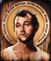# Why does spatial join on geodataframes return empty result?

713
0
07-08-2019 10:52 AMOccasional Contributor II

I have two `geodataframes` that I want to perform a spatial join on; one is points, one is polygons. They are both `dtype: int64`. They both have `.crs = {'init': 'epsg:4326'}`. I have verified both of them in `ArcMap` that the points are indeed within the polys. I even performed an intersect between these points and polygons in `ArcMap` and it produced the correct new feature class (I have reasons for wanting to do this without `arpy`/manually in ArcMap). **Note: the points are from a shapefile and the polys are from a feature classHowever, when I turn my centroid points and parcel polygons into `geodataframes` and use `.sjoin()`, it returns an empty `geodataframe`. I want the output to be a point-based geometry so that I can eventually turn it back into a point shapefile. I have tried:

``intersect_test = gpd.sjoin(cents, polys, how='left', op='intersects')``

and

``intersect_test = gpd.sjoin(polys, cents, how='inner', op='intersects')``

and

``intersect_test = gpd.sjoin(polys, cents, how='right', op='intersects')``

and

``intersect_test = gpd.sjoin(cents, polys, how='left', op='intersects')``

and pretty much every other configuration I can think of and it either returns the data for one of them, or returns a completely empty geodataframe result.

`` intersect_test = gpd.sjoin(cents, polys, how='inner', op='intersects') intersect_test.count()Out:  BLD_UNITS       0LAND_USE_T      0PARCEL_ID       0PROP_IND_T      0STORY_NBR       0geometry        0index_right     0GEOID           0CensusPop       0CBArea          0ST_FIPS         0Shape_Length    0Shape_Area      0CO_FIPS         0HU_Pop          0Sq_Ft           0dtype: int64``

How can I resolve this without having to manually perform the intersect in ArcMap?

EDIT Here's how I made my `geodataframes`. As I mentioned; one is a shapefile and one is a `.gdb feature class`. Question: Could this be due to projection/`crs`-related problem?

``#create poly gdfcb_gdb = r"C:\Projects\Pop_Alloc\CensusBlocksStates.gdb"cb_feat = "CBs_{}".format(state)cents = gpd.read_file(cb_gdb, layer=cb_feat)cents.crs = {'init': 'epsg:4326'}#create point gdf. The parcel centroids are first created from a polygon gdf. The new gdf is written to a shapefile to be tested against the census  block polygons (to make sure they do in fact fall within the boundaries of  the census blocks (cbs)) and the new centroid gdf(its a `type='geopandas.geodataframe.GeoDataFrame'`) is then used in the `.sjoin`        cents = parcel_res_df cents['geometry'] =  cents['geometry'].representative_point()cents_out_feat = r"C:\Projects\Pop_Alloc\{}_Res_centroids.shp".format(state)cents.crs = {'init': 'epsg:4326'}cents.to_file(cents_out_feat)``
Tags (4)
0 Replies